## PySparkShell application UI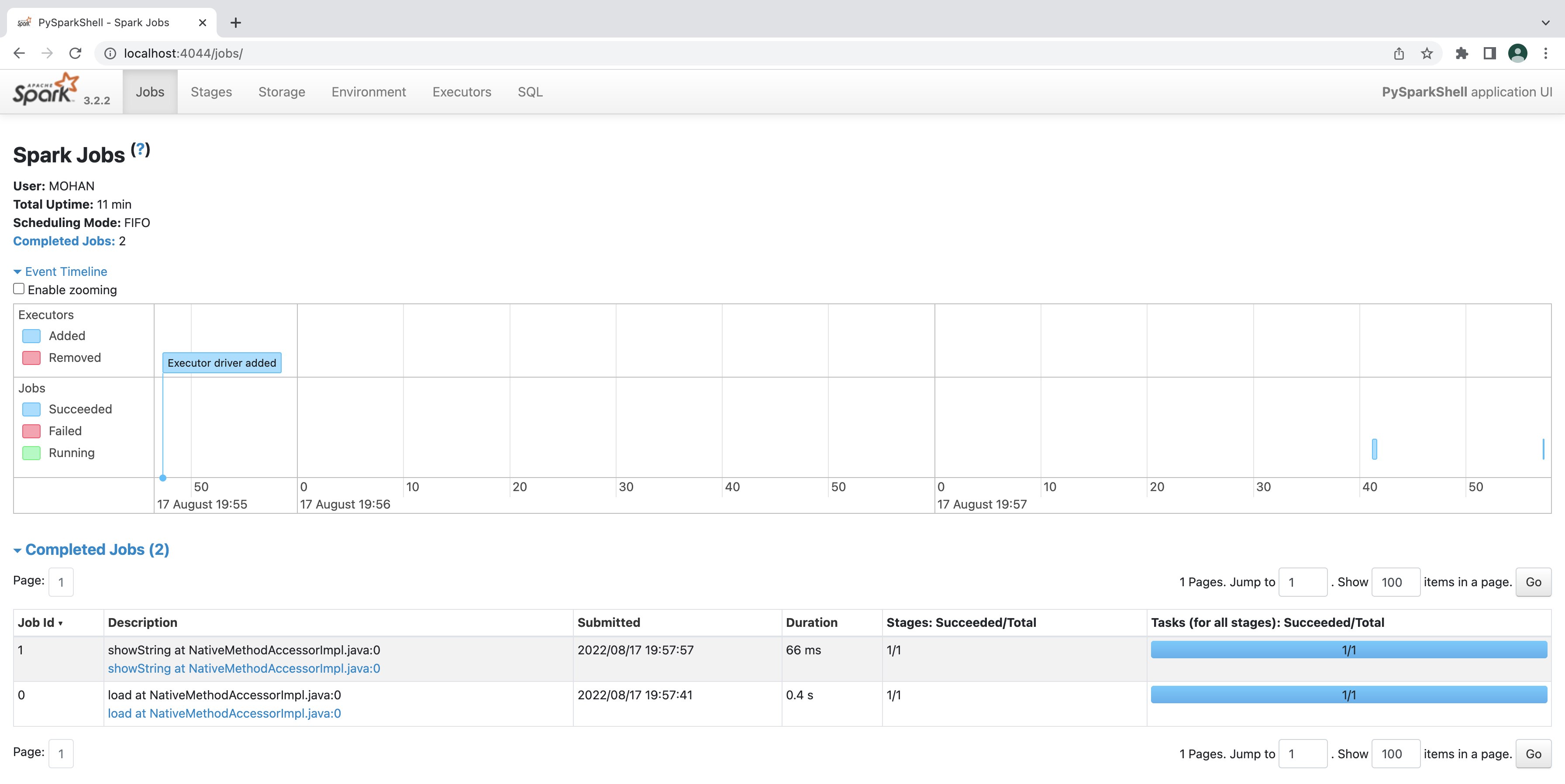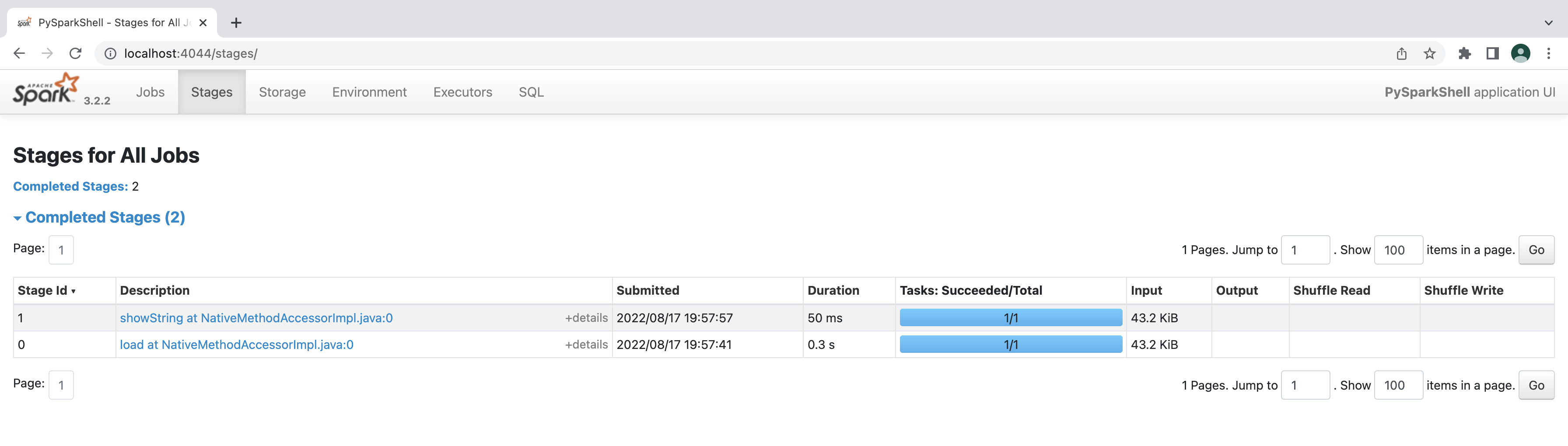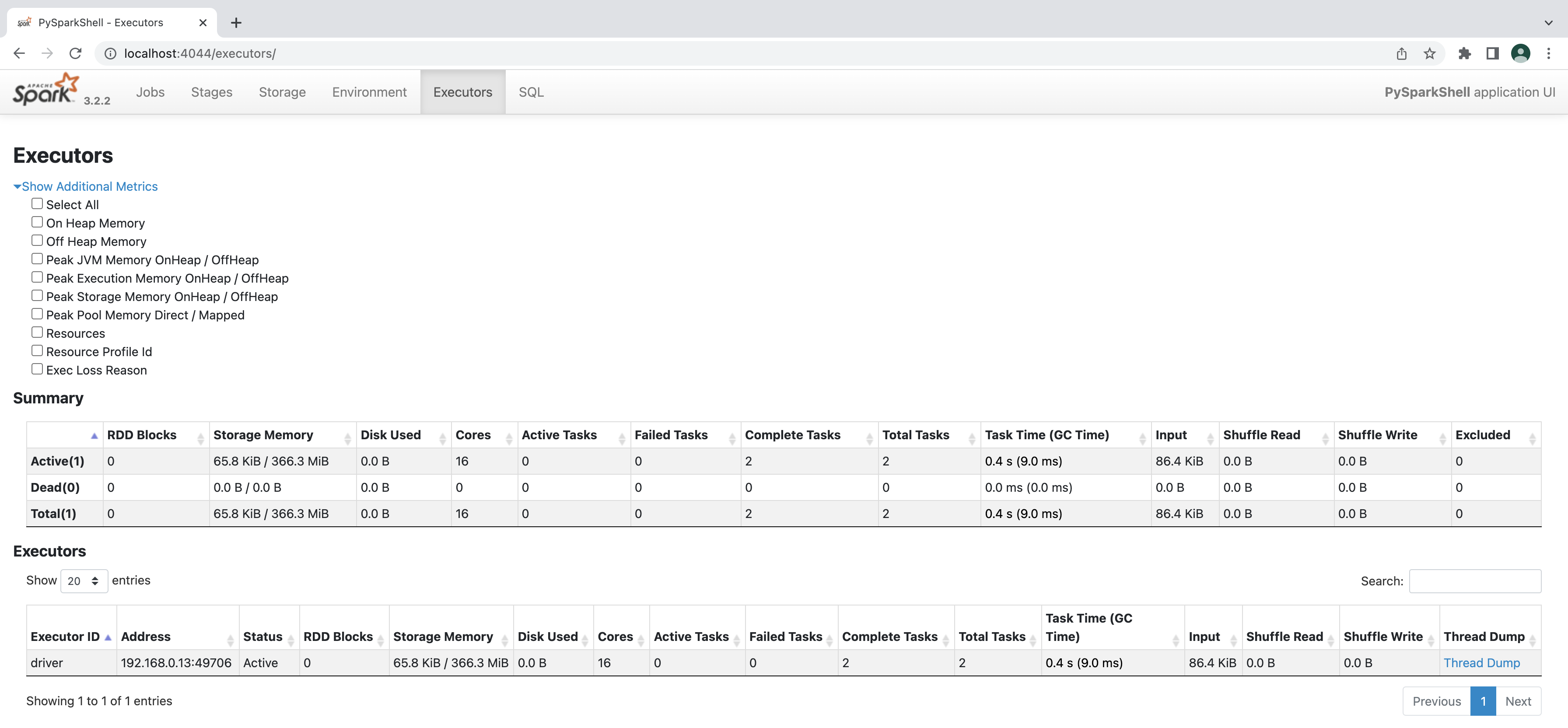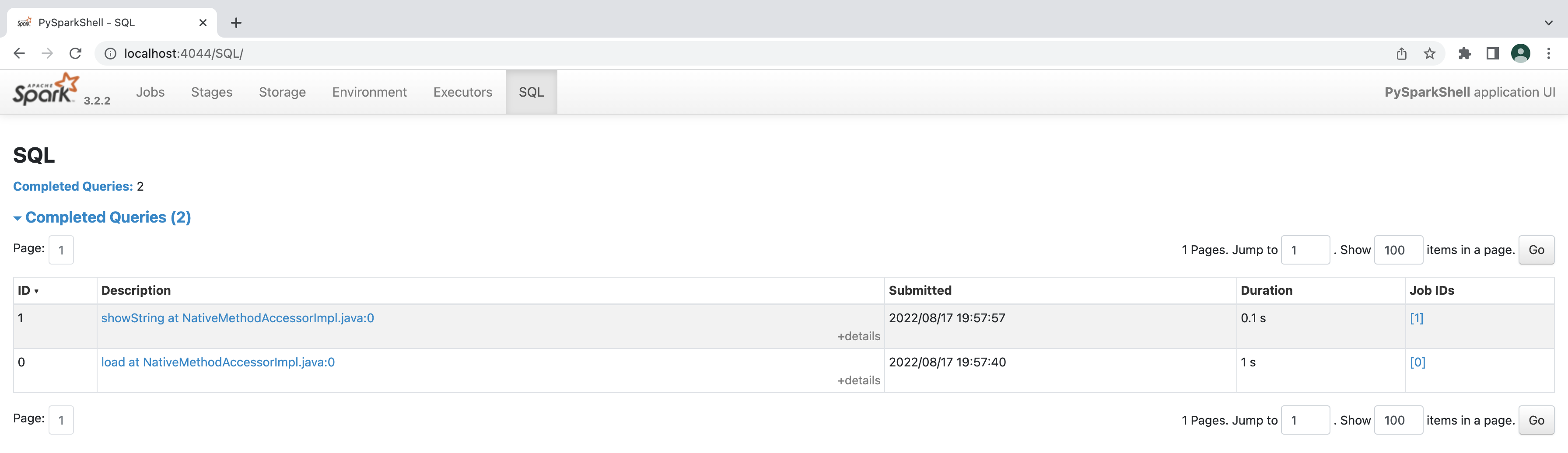## Simple linear regression

y = mx + c

y – Dependent variable(DV)

x – Independent variable(IV)

c – constant

## Filling missing data using scikit

from sklearn.impute import SimpleImputer
We are importing the simple imputer from scikit library.
imputer = SimpleImputer(missing_values=np.nan, strategy=’mean’)
The strategy used is mean, where the missing data is replaced by average of the values.
imputer.fit(X[:, 1:3])
The number of columns on which the above function is used is 2 i.e between 1 and 3.
X[:, 1:3] = imputer.transform(X[:, 1:3])
The new column is again assigned to the 2 columns.

## Importing dataset in Python

x = dataset.iloc[:,:-1].values

y = dataset.iloc[:, -1].values

In the above lines, read_csv and iloc are functions.

[:,:-1] is slicing range of the values.

Basic operations

```a=10
b=5

sub = a-b
mul = a*b
div = a/b
mod = a%b

print(sub)
print(mul)
print(div)
print(mod)```

## Python Post for the day – 6th October 2019

Simple Python Program

`print('python is big!')`

## Functions and Built-in Functions in Python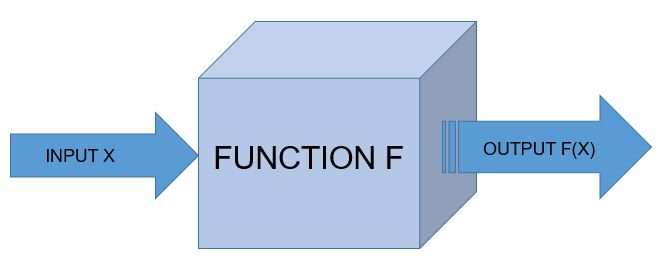Function

A function is the simplest callable object in Python.

NOTE: For Indentation either use TAB space or 4 space characters, never both.

def tax(bill):
“””Adds 25% tax to a restaurant bill.”””
bill *= 1.25
print “With tax: %f” % bill
return bill

def tip(bill):
“””Adds 35% tip to a restaurant bill.”””
bill *= 1.35
print “With tip: %f” % bill
return bill

meal_cost = 100
meal_with_tax = tax(meal_cost)
meal_with_tip = tip(meal_with_tax)

Function Syntax

“”” This is spam function”””            /* Comment*/
print “Spaceship!”                            /*body*/

spam()

Call and Response

def square(n):
“””Returns the square of a number.”””
squared = n**2
print “%d squared is %d.” % (n, squared)
return squared

square(5)

Parameters and Arguments

A function can require as many parameters as you’d like, but when called the function, it should generally pass in a matching number of arguments.

result = base**exponent
print “%d to the power of %d is %d.” % (base, exponent, result)

Functions Calling Functions

A function can call another function.

def n_plus_one(n):
return n + 1

return n_plus_one(n) + 2

Importing Module

A module is a file that contains definitions, including variables and functions, that we can use once it is imported.

import math
print math.sqrt(25)

math includes a number of useful variables and functions, and sqrt() is one of those functions. In order to access math, all you need is the import keyword. When you simply import a module this way, it’s called a generic import.

It’s possible to import only specific variables or functions from a given module. Pulling in just a single function from a module is called a function import.

# Import *just* the sqrt function from math

from math import sqrt

if we want all the variables and functions in a module but don’t want to have to constantly type math then we can use

# Import *everything* from the math module

from math import *

Built-in Functions

max()

The max() function takes any number of arguments and returns the largest one.

>> maximum = max(10,35,64)
>> print maximum

min()

min() function returns the smallest of a given series of arguments.

>> minimum = (10,35,64)
>> print minimum

abs()

The abs() function returns the absolute value of the number it takes as an argument, it gives the number’s distance from 0 on an imagined number line.
>> absolute = abs(-42)
>> print absolute

type()

The type() function returns the type of the data it receives as an argument.

>> print type(42)
>> print type(4.2)
>> print type(‘spam’)

Now let us see the code which returns biggest, smallest and absolute values.

def biggest_number(*args):
print max(args)
return max(args)

def smallest_number(*args):
print min(args)
return min(args)

def distance_from_zero(arg):
print abs(arg)
return abs(arg)

biggest_number(-105, -53, 51, 100)
smallest_number(-106, -55, 54, 105)
distance_from_zero(-104)

These are some of the functions and built-in functions in python.

## Basics of Python – Part 2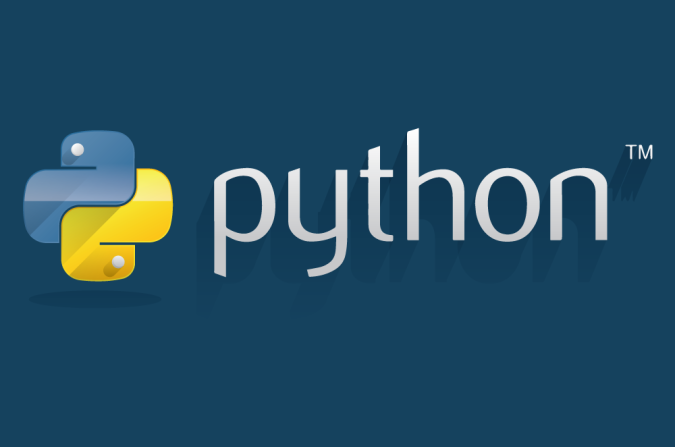Dot Notation

Using dot notation with strings.
Let us consider an example where we convert the string to upper case using dot notation.

>>> mysore = “The city is awesome !”

>>> print len(mysore)
>>> print mysore.upper()

Printing strings

>>> print(“This String”)

Printing Variables

>>> Stone_cold = “Stunner”
>>> print(Stone_cold)

Concatenation

Combining strings together is called concatenation . The + operator combine the strings.

>>> print “Indian “+ “Air ” +”Force ”

The space after the word is to make the string look like sentence.

String Conversion

The str() method converts non-strings into strings.

>>> print “Sourav Ganguly scored “+ str(100) + “on his Test Debut”

String Formatting

The % operator after a string combines a string with variables. The % operator will replace  %s in the string with the string variable.

Example 1:
>>> string_1 = “Sourav”

>>> print “Indian captain %s is also called as %s.” % (string_1, string_2)

Example 2:

>>> batsman = raw_input(“Who is the batsman?”)
>>> captain = raw_input(“Who is the captain?”)
>>> series = raw_input(“Which series did he win for India?”)

>>> print “The Indian %s and %s Sourav Ganguly won %s series for India.” % (batsman, captain, series)

Date and Time

We can import datetime function from datetime module.

>>> from datetime import datetime

Current Date and Time

Here is the code to print current date and time.

>>> from datetime import datetime
>>> now = datetime.now()
>>> print datetime.now()

Control Flow

The ability to choose from different situations based on what’s happening in the program.

>>> x = int(input(“Please enter an integer: “))

>>> if x < 0:

…     x = 0

…     print(‘Negative changed to zero’)

… elif x == 0:

…     print(‘Zero’)

… elif x == 1:

…     print(‘Single’)

… else:

…     print(‘More’)

if statements, for statements, range fuction, break and continue, else clause loops, pass statements are few of the control flow statements.

Comparators

Equal to (==)
Not equal to (!=)
Less than (<)
Less than or equal to (<=)
Greater than (>)
Greater than or equal to (>=)

Boolean Operators

AND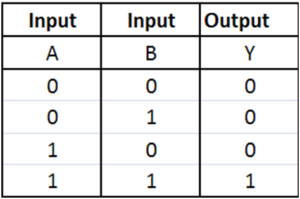OR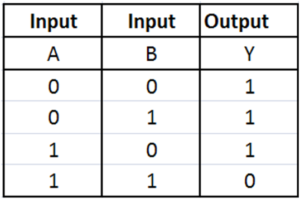NOT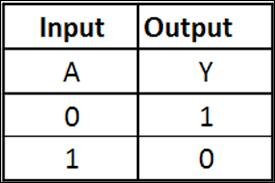Conditional Syntax

The statements used for decision making are called conditional statements.

if condition_1:

statement_block_1

elif condition_2:

statement_block_2

else:

statement_block_3

This post concludes basics of python.

## Basics of Python – Part 1Download the python interpreter from this link . Choose either 3.0 or 2.0 version depending upon your compatibility. In this exercise version 2.0 was used for examples. Based on the current and future trends I would recommend 3.0.

Now let us look into some basic concepts in python programming language.

Variables:

As with most programming languages, assigning a value for a variable is as simple as:
a = 10

Booleans:

The two switch states True or False can be assigned to a variable.
a = True
(or)
a = False

Reassigning variable values:

A variable b = 10 can be given a value as b = 5 in the next line, and the value can be printed using
print b

Indentation:

Indentation is one of the most important aspect of python, either use a Tab space or 4 white spaces but not both. Here’s an example of a simple python function.

def example()
var = 15
return var
print example()

Single-line Comment:

Use # at the beginning of a line to give a single line comment.
# This is a single line comment in python.

If the comment is more than one line use double inverted comma’s thrice at the start and end of the comment.
“”” This is a multi-line
comment”””

Basic Math Operations:

All basic math operations such as addition(+), subtraction(-), multiplication(*) and division(%) can be performed similar to other programming languages.
>>print 36 + 64
100
>>print 200 – 100
100
>>print 10 * 10
100
>>print 1000 / 10
100

Exponentiation:

In python, the function of exponential can be performed using ** keys.
>> chairs = 10 ** 2
>> print chairs
100

Modulo:

Modulo operation can be performed using %.

Strings:

Assigning strings values and printing them.
python = “Hello world!”
print(“python”)

Escaping characters:

In python both single quotes and double quotes can be used for commenting. When using single quote, the interpreter doesn’t understands the code. In such cases we can use escape characters such as backslash(\).
‘Isn\’t it the python?’

Accessing by Index:

We can access any character of a string by indexing. Indexing starts from 0.
>>sixth_letter = “python”
>>print sixth_letter
n

String methods:

We can calculate length of the string using len()
>>python = “Indian”
>>len(python)
>>print len(python)
6

We can convert lower case string to upper case string
>>python = “indian”
>>”indian”.upper()
>>print python.upper()
INDIAN

We can convert upper case string to lower case string
>>python = “INDIAN”
>>”INDIAN”.lower()
>>print python.lower()
indian

We can convert a variable to a string
>>pi = 3.14
>>print str(pi)
3.14

So, this concludes basics of python part-1.